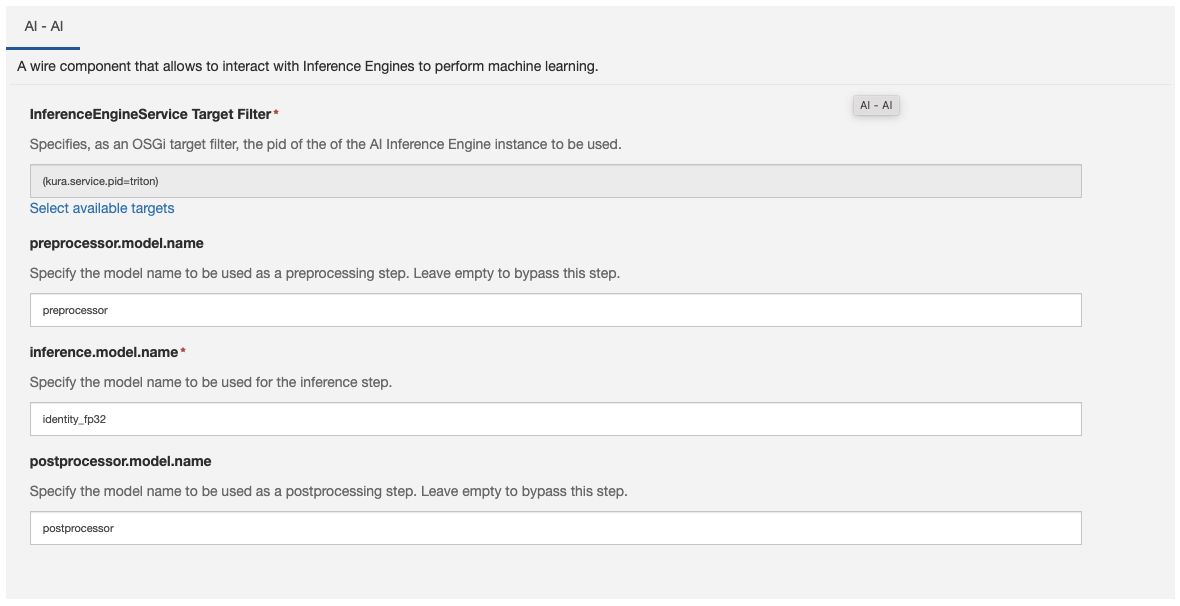# AI Wire Component

The component allows interacting with an `InferenceEngineService` to perform machine learning-related operations.

An InferenceEngineService is a Kura service that implements a simple API to interface with an Inference Engine. The Inference Engine allows to perform inference on trained Artificial Intelligence models commonly described by a file and some configuration for explaining its input and outputs. An example of Inference Engine implementation is the Nvidia™ Triton Server inference engine.

In a normal machine learning flow, the input is preprocessed before it is given to the machine learning algorithm, and the result is processed again to be adapted to the rest of the pipeline.

Once these models are loaded in the engine, the AI wire component allows to specify the name of the models that are used in the pre-processing, infer, and post-processing steps. Only the infer model name is mandatory so that it is possible to just use the strictly necessary steps in case the pre/post-processing is performed directly by the infer step.## Models Input and Output formats

The AI wire component takes a `WireEnvelope` as an input, it processes its records and feeds them to the specified preprocessing or inference model. The outputs of the inference or the post-processing step are then reconverted into a wire record. This section explains the inputs and output formats that the wire component is expecting. Not specifying the models according to this contract will result in a non-functioning inference.

The 3 inference steps are applied on each `WireRecord` contained in the input `WireEnvelope`.

The inputs and outputs will have assigned the corresponding Kura `DataType`, which can be one of:

• `BOOLEAN`
• `DOUBLE`
• `FLOAT`
• `INTEGER`
• `LONG`
• `STRING`
• `BYTE_ARRAY`

Reference to Introduction for the data types that are allowed to flow through the wires.

The models that manage the input and the output must expect a list of inputs such that:

• each input corresponds to an entry of the `WireRecord` properties
• the entry key will become the input name (e.g. in the case of an asset, the channel name becomes the tensor name)
• input shape will be ``

In the following, two example configurations for Triton Inference Engine models are provided. A complete usage example that implements an Anomaly Detector using a RaspberryPi SenseHat is provided in the Kura examples repository.

### Input Specification Example

Following, an example of a model configuration for the Nvidia™ Triton Inference Engine. It expects the input from the `WireEnvelope` that contains a record with properties:

• `ACCELERATION` of type `Float`
• `CHANNEL_0` of type `Integer`
• `STREAM` of type `byte[]`
• `GYRO` of type `Boolean`

This record can be generated from an asset with channel names as above. The output will be a single tensor of type `Float`, of shape 1x13, and name `OUT_PRE`.

Note that each input will have shape 1.

``````name: "preprocessor"
backend: "python"

input [
{
name: "ACCELERATION"
data_type: FP32
dims: [ 1 ]
}
]
input [
{
name: "CHANNEL_0"
data_type: INT32
dims: [ 1 ]
}
]
input [
{
name: "STREAM"
data_type: BYTES
dims: [ 1 ]
}
]
input [
{
name: "GYRO"
data_type: BOOL
dims: [ 1 ]
}
]
output [
{
name: "OUT_PRE"
data_type: FP32
dims: [ 13 ]
}
]
instance_group [{ kind: KIND_CPU }]
``````

### Output Specification Example

Following, an example of a Nvidia™ Triton Inference Engine configuration that takes input `IN_POST` and produces outputs that will be mapped to a `WireRecord` with the properties as follows:

• `RESULT0` of type `Boolean`
• `RESULT1` of type `Integer`
• `RESULT2` of type `byte[]`
• `RESULT3` of type `Float`

Note that each output will have shape 1.

``````name: "postprocessor"
backend: "python"

input [
{
name: "IN_POST"
data_type: FP32
dims: [ 1, 5 ]
}
]
output [
{
name: "RESULT0"
data_type: BOOL
dims: [ 1 ]
}
]
output [
{
name: "RESULT1"
data_type: INT32
dims: [ 1 ]
}
]
output [
{
name: "RESULT2"
data_type: BYTES
dims: [ 1 ]
}
]
output [
{
name: "RESULT3"
data_type: FP32
dims: [ 1 ]
}
]
instance_group [{ kind: KIND_CPU }]
``````